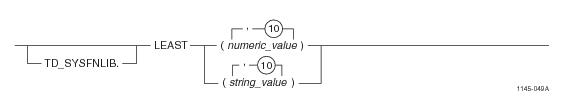# 15.00 - LEAST - Teradata Database

## Teradata Database SQL Functions, Operators, Expressions, and Predicates

Product
Release Number
15.00
Content Type
Programming Reference
Publication ID
B035-1145-015K
Language
English (United States)

## LEAST

### Purpose

Returns the least value among a list of numeric_values or string_values.

### Syntaxwhere:

 Syntax element … Specifies … TD_SYSFNLIB the name of the database where the function is located. numeric_value a numeric argument. LEAST supports 1-10 numeric values. If numeric_value is the data type of the first argument, the return data type is numeric. The remaining arguments in the input list must be the same or compatible types. If either input parameter is NULL, NULL is returned. string_value a character argument. LEAST supports 1-10 string values. If string_value is the data type of the first argument, the return data type is string. The remaining arguments in the input list must be the same or compatible types. String comparison uses non-padded comparison semantics. Character comparison is binary and based on the numerical codes of the characters and the string is treated as a sequence of bytes for the comparison rather than character by character. If either input parameter is NULL, NULL is returned.

### ANSI Compliance

This is a Teradata extension to the ANSI SQL:2011 standard.

### Invocation

LEAST is an embedded services system function. For information on activating and invoking embedded services functions, see “Embedded Services System Functions” on page 24.

### Argument Types and Rules

Expressions passed to this function must have the following data types:

• numeric_value = BYTEINT, SMALLINT, INTEGER, BIGINT, DECIMAL/NUMERIC, FLOAT/REAL/DOUBLE PRECISION, or NUMBER
• string_value = CHAR or VARCHAR
• ### Result Type

LEAST is a scalar function whose return value data type depends upon the data type associated with the numeric_value or string_value passed to the function.

• If numeric_value is a numeric data type, LEAST determines which argument has the highest precedence, converts the other arguments to that data type, and returns that data type. For details about the order of precedence, see “Compatible Types” in SQL External Routine Programming.
• If the data type is DECIMAL/NUMERIC and the precision and scale of the input arguments are different, the precision and scale of the return data types are set to achieve the maximum precision possible. For example, if the input arguments are DECIMAL(6,3), DECIMAL(7,4), and DECIMAL(8,7), the return data type would need 3 digits to the left of the decimal point and 7 digits to the right of the decimal point to avoid any reduction in precision. In this case, the return data type is set to DECIMAL(10,7).

In cases where it is not possible to maintain the maximum precision, the data is rounded according to the DBS Control Record RoundHalfWayMagUp field. For example, if the input arguments are DECIMAL(32, 8) and DECIMAL(30, 28), the return data type is DECIMAL(38,14). This allows for 24 digits to the left of the decimal point (required for the DECIMAL(32,8) parameter), and 14 digits to the right of the decimal point. If the DECIMAL(30,28) input argument is the least value, it is rounded to 14 places to the right of the decimal point.

If the data type is fixed point NUMBER and the precision is less than or equal to 38, the precision and scale of the return type are calculated with the same method used for DECIMAL/NUMERIC. However, if the precision is greater than 38, the return type is changed to NUMBER(*) to avoid loss of accuracy. If the data type is floating point NUMBER, the return type is NUMBER(*).

• If string_value is a character data type, LEAST converts the 2nd through 10th argument to the data type of the 1st argument and returns values as VARCHAR in the character set of the first argument.
• ### Example

The following query returns 6.

`SELECT LEAST(13, 6); `

### Example

In the following query, if the input arguments have data types of DECIMAL(5,4) and DECIMAL(4,2), the return data type is DECIMAL(6,4) and the return value is 1.1234.

`SELECT LEAST(1.1234, 36.12); `

### Example

The following query returns 'alpha'.

`SELECT LEAST('apples', 'alpha'); `